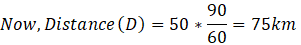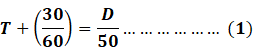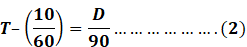Question of The Day13-02-2020

If a person covers a certain distance at 50 km/hr he got late by half an hour but when he covers the same distance at 90 km/hr he reaches 10 minutes early. Find the distance (in km) he travels in each case?

Correct Answer : b ) 75

Explanation :

Quick Approach

We know that

Distance = Speed * Time

For the constant distance, we can say that speed is inversely proportional to the time period

Thus, according to the question the ratio of the speeds is given as 50 : 90 i.e., 5 : 9

Now, the ratio of the time periods covering the same distance with the speeds 50 km/hr and 90 km/hr respectively will be 9 : 5

Let us assume that time period in covering the distance at 50 km/hr is 9x and that at 90km/hr is 5x

Now, according to question we can say that

9x – 5x = 40 minutes

x = 10 minutes

Thus, the time required to cover the distance at 50 km/hr will be 90 minutesHence, (b) is the correct answer.

Basic Approach

Let the distance travelled be D km

And, let the usual time to cover the distance D km be T hours.

As, we know

Distance=Speed   *   Time

Here, the time required to cover the distance D at 50 km/hr will beAnd, time required to cover the distance D km at 90 km/hr beSolving equations (1) and (2) we get,

D = 75 km

Hence, (b) is the correct answer.

Such type of question is asked in various government exams like SSC CGL, SSC MTS, SSC CPO, SSC CHSL, RRB JE, RRB NTPC, RRB GROUP D, RRB OFFICER SCALE-I, IBPS PO, IBPS SO, RRB Office Assistant, IBPS Clerk, RBI Assistant, IBPS RRB OFFICER SCALE 2& 3, UPSC CDS, UPSC NDA etc.

Read Daily Current Affairs, Banking Awareness, Hindi Current Affairs, Word of the Day, and attempt free mock tests at PendulumEdu and boost your preparation for the actual exam.0